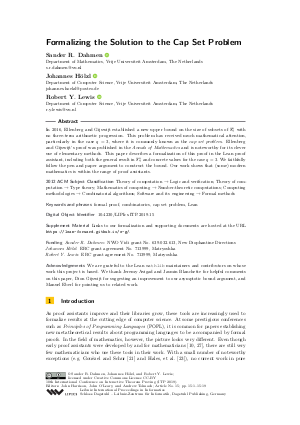Document# Formalizing the Solution to the Cap Set Problem

### Authors Sander R. Dahmen, Johannes Hölzl, Robert Y. Lewis## File

LIPIcs.ITP.2019.15.pdf
• Filesize: 0.51 MB
• 19 pages

## Acknowledgements

We are grateful to the Lean mathlib maintainers and contributors on whose work this project is based. We thank Jeremy Avigad and Jasmin Blanchette for helpful comments on this paper, Dion Gijswijt for suggesting an improvement to our asymptotic bound argument, and Manuel Eberl for pointing us to related work.

## Cite As

Sander R. Dahmen, Johannes Hölzl, and Robert Y. Lewis. Formalizing the Solution to the Cap Set Problem. In 10th International Conference on Interactive Theorem Proving (ITP 2019). Leibniz International Proceedings in Informatics (LIPIcs), Volume 141, pp. 15:1-15:19, Schloss Dagstuhl - Leibniz-Zentrum für Informatik (2019)
https://doi.org/10.4230/LIPIcs.ITP.2019.15

## Abstract

In 2016, Ellenberg and Gijswijt established a new upper bound on the size of subsets of F^n_q with no three-term arithmetic progression. This problem has received much mathematical attention, particularly in the case q = 3, where it is commonly known as the cap set problem. Ellenberg and Gijswijt’s proof was published in the Annals of Mathematics and is noteworthy for its clever use of elementary methods. This paper describes a formalization of this proof in the Lean proof assistant, including both the general result in F^n_q and concrete values for the case q = 3. We faithfully follow the pen and paper argument to construct the bound. Our work shows that (some) modern mathematics is within the range of proof assistants.

## Subject Classification

##### ACM Subject Classification
• Theory of computation → Logic and verification
• Theory of computation → Type theory
• Mathematics of computing → Number-theoretic computations
• Computing methodologies → Combinatorial algorithms
• Software and its engineering → Formal methods
##### Keywords
• formal proof
• combinatorics
• cap set problem
• Lean

## Metrics

• Access Statistics
• Total Accesses (updated on a weekly basis)
0